Get instant live expert help with Excel or Google Sheets“My Excelchat expert helped me in less than 20 minutes, saving me what would have been 5 hours of work!”

#### Post your problem and you’ll get Expert help in seconds.

Your message must be at least 40 characters
Our professional Expert are available now. Your privacy is guaranteed.

# Backwards VLOOKUP: A Quick Guide with Examples

The VLOOKUP function is very powerful. However, it is limited to only referencing data that is in the leftmost column of the table array. This tutorial will step through a simple alternative to VLOOKUP which allows for a reverse lookup

## Final Formula

`=INDEX(A3:B8,MATCH(E2,B3:B8,0),1)`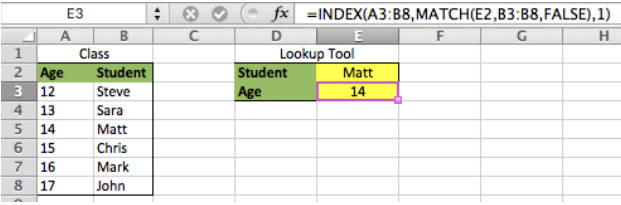Figure 1. Backwards VLOOKUP

## Setting up the Data

First, we set our data up so that the value to be returned is in Column A and the lookup array is in Column B. Then, we will put the lookup value in cell E2 and we will use cell E3 as our formula cell where we want the data to be returned

• In this example, we will lookup the student’s age based on his/her name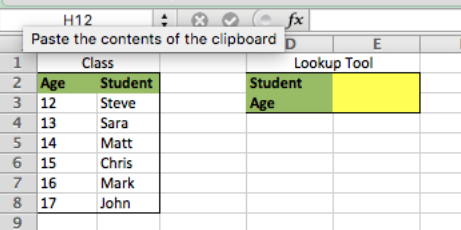Figure 2. Backwards VLOOKUP

Now, we have our data setup, but before we will get into the formula, we will first breakdown the different components of the formula

## INDEX MATCH Method

The INDEX and MATCH functions our two individual functions that on their own, aren’t very powerful. We will first walk through each function and then we will merge them together in order to create a super lookup function

• The INDEX function allows a user to specify where in table he/she would like to return data. To do this, he/she must specify where the table is located and the ROW and COLUMN within the table that he/she wants to return
• INDEX Syntax: =Index(Table Array, ROW, COLUMN)
• In cell E2, type this formula =INDEX(A3:B8,3,2)”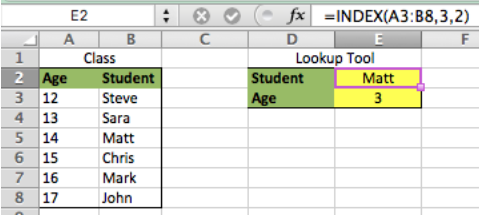Figure 3. Backwards VLOOKUP

In this Example we see that the formula returns “Matt” because his name is located in the third row and second column of the table

• The MATCH function allows a user to return the ROW within a table array where a match to the lookup value is found
• MATCH Syntax = MATCH(Lookup Value, Table Array, Approximate Match [True/False])
• In cell E3, type this formula =MATCH(E2,B3:B8,FALSE)”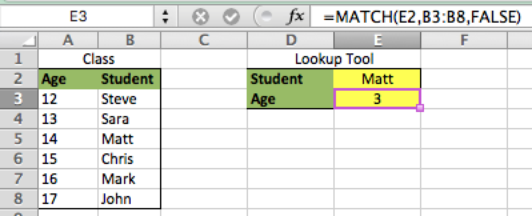Figure 4. Backwards VLOOKUP

In this Example we see that the formula returns “3” because ROW 3 is where the matching value to cell E2 is in the lookup array. Next, we will merge the INDEX and MATCH functions to complete the process

## Putting it all Together

We can now use the INDEX function to tell Excel where to lookup the value and the MATCH function to tell the INDEX function which row to find the lookup value in the lookup array To do this, we nest the MATCH formula from the above example inside the INDEX formula from the earlier example. However, we change the column to “1 “instead of “2” since we want to return the age of the student

• In cell E3 type this formula =INDEX(A3:B8,MATCH(E2,B3:B8,FALSE),1)”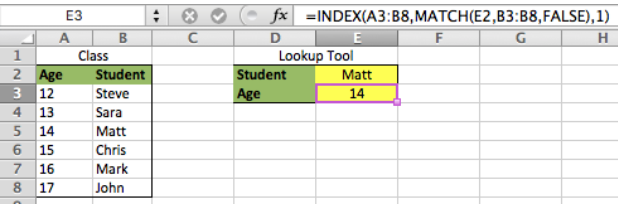Figure 5. Backwards VLOOKUP

We can see in this Example that the formula in cell E3 returns “14” because “Matt” is located in the third ROW of the table array and his age is “14”

Are you still looking for help with the VLOOKUP function? View our comprehensive round-up of VLOOKUP function tutorials here.

### Did this post not answer your question? Get a solution from connecting with the expert.Another blog reader asked this question today on Excelchat:
Solution examplesHow can I automatically change different cells and the information they display if we select different option from a drop down list?
Solved by V. U. in 59 minsindex and match with duplicates. I need to use another column as a reference, so my return value has two match the value of two things for it to return
Solved by E. H. in 60 minsI have a file with two sheets. On the 1st, I have dollars in O13and in O16 a number signifying 1-6 people. On the second workbook is a matrix that corresponds to the dollar amount AND the number of people. For example, sheet 1 O13 is \$6,000. O16 is 3. I want a formula to find \$6,000 (not higher) on sheet 2 in column A. Then I want it to compare sheet 1 O16 to the correct column (B-G representing 1-6) on sheet 2 for an exact match to place in sheet1 O17. The range of sheet1 O13 is referenced on sheet2 A5-A383, and sheet 1 O16 is referenced on sheet2 B5:G383. I have an INDEX Match but it isn't quite working, yet.
Solved by B. B. in 60 minsI need a formula to calculate the MAX of a range of 167 rows in column D, then find the MAX of the next 167 rows in column D and so on continuously through the entire sheet. Example MAX D2:D169, D170:D337, D338:D505 etc...
Solved by I. Q. in 60 minsI have two lists. One is a list of part numbers. The other is a list of long text descriptions. I can use VLOOKUP with "*"&cell address"*" to find the first match. But how can I find the second and third matches? I have tried using INDEX and SHORT but the wild cards no longer find the match like they do in VLOOKUP. Can you help?
Solved by S. J. in 36 mins## Subscribe to Excelchat.coAnother blog reader asked this question today on Excelchat: